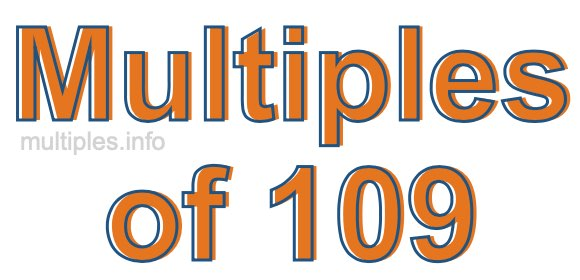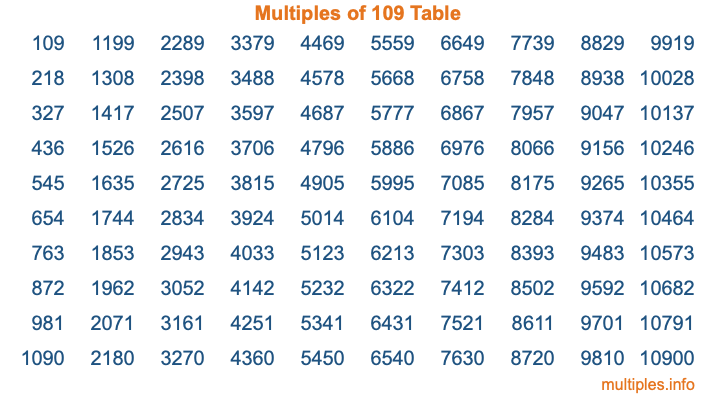Multiples of 109Welcome to the Multiples of 109 page. Here we will first teach you everything you will ever need to know about the multiples of 109, and then give you a study guide summary of everything we taught you to make sure you remember it all. Use this page to look up facts and learn information about the multiples of 109. This page will make you a multiples of one hundred nine expert!

Definition of Multiples of 109
Multiples of 109 are all the numbers that when divided by 109 equal an integer. Each of the multiples of 109 are called a multiple. A multiple of 109 is created by multiplying 109 by an integer.

Therefore, to create a list of multiples of 109, you start with 1 multiplied by 109, then 2 multiplied by 109, then 3 multiplied by 109, and so on for as long as you want. Thus, the list of the first five multiples of 109 is 109, 218, 327, 436, and 545. To see a larger list of multiples of 109, see the printable image of Multiples of 109 further down on this page. We also have a category where you can choose any nth multiple of 109.

Multiples of 109 Checker
The Multiples of 109 Checker below checks to see if any number of your choice is a multiple of 109. In other words, it checks to see if there is any number (integer) that when multiplied by 109 will equal your number. To do that, we divide your number by 109. If the the quotient is an integer, then your number is a multiple of 109.

Is  a multiple of 109?

Least Common Multiple of 109 and ...
A Least Common Multiple (LCM) is the lowest multiple that two or more numbers have in common. This is also called the smallest common multiple or lowest common multiple and is useful to know when you are adding our subtracting fractions. Enter one or more numbers below (109 is already entered) to find the LCM.

Check out our LCM Calculator if you need more details about the Least Common Multiple or if you need the LCM for different numbers for adding and subtraction fractions.

nth Multiple of 109
As we stated above, 109 is the first multiple of 109, 218 is the second multiple of 109, 327 is the third multiple of 109, and so on. Enter a number below to find the nth multiple of 109.

th multiple of 109

Multiples of 109 vs Factors of 109
109 is a multiple of 109 and a factor of 109, but that is where the similarities end. All postive multiples of 109 are 109 or greater than 109. All positive factors of 109 are 109 or less than 109.

Below is the beginning list of multiples of 109 and the factors of 109 so you can compare:

Multiples of 109: 109, 218, 327, 436, 545, etc.

Factors of 109: 1, 109

As you can see, the multiples of 109 are all the numbers that you can divide by 109 to get a whole number. The factors of 109, on the other hand, are all the whole numbers that you can multiply by another whole number to get 109.

It's also interesting to note that if a number (x) is a factor of 109, then 109 will also be a multiple of that number (x).

Multiples of 109 vs Divisors of 109
The divisors of 109 are all the integers that 109 can be divided by evenly. Below is a list of the divisors of 109.

Divisors of 109: 1, 109

The interesting thing to note here is that if you take any multiple of 109 and divide it by a divisor of 109, you will see that the quotient is an integer.

Multiples of 109 Table
Below is an image of the first 100 multiples of 109 in a table. The table is in chronological order, column by column. The first column has the first ten multiples of 109, the second column has the next ten multiples of 109, and so on.The Multiples of 109 Table is also referred to as the 109 Times Table or Times Table of 109. You are welcome to print out our table for your studies.

Negative Multiples of 109
Although not often discussed or needed in math, it is worth mentioning that you can make a list of negative multiples of 109 by multiplying 109 by -1, then by -2, then by -3, and so on, to get the following list of negative multiples of 109:

-109, -218, -327, -436, -545, etc.

Multiples of 109 Summary
Below is a summary of important Multiples of 109 facts that we have discussed on this page. To retain the knowledge on this page, we recommend that you read through the summary and explain to yourself or a study partner why they hold true.

There are an infinite number of multiples of 109.

A multiple of 109 divided by 109 will equal a whole number.

109 divided by a factor of 109 equals a divisor of 109.

The nth multiple of 109 is n times 109.

The largest factor of 109 is equal to the first positive multiple of 109.

109 is a multiple of every factor of 109.

109 is a multiple of 109.

A multiple of 109 divided by a divisor of 109 equals an integer.

109 divided by a divisor of 109 equals a factor of 109.

Any integer times 109 will equal a multiple of 109.

Multiples of a Number
Here you can get the multiples of another number, all with the same attention to detail as we did for multiples of 109 on this page.

Multiples of
Multiples of 110
Did you find our page about multiples of one hundred nine educational? Do you want more knowledge? Check out the multiples of the next number on our list!